# Auto-Interval Aggregation Processor

Modified on Tue, 30 Nov 2021 at 01:07 PM

The Auto-Interval Aggregation Processor aggregates a dataset based on a timestamp or ratio-scaled column. Depending on the number of cuts to be computed, the aggregation interval varies automatically.

An example can be seen in the following picture: the timestamp or ratio-scaled column defines an overall interval, which is then divided by the defined cuts into several intervals. With two cuts to be had, the overall interval is divided into three sub-intervals.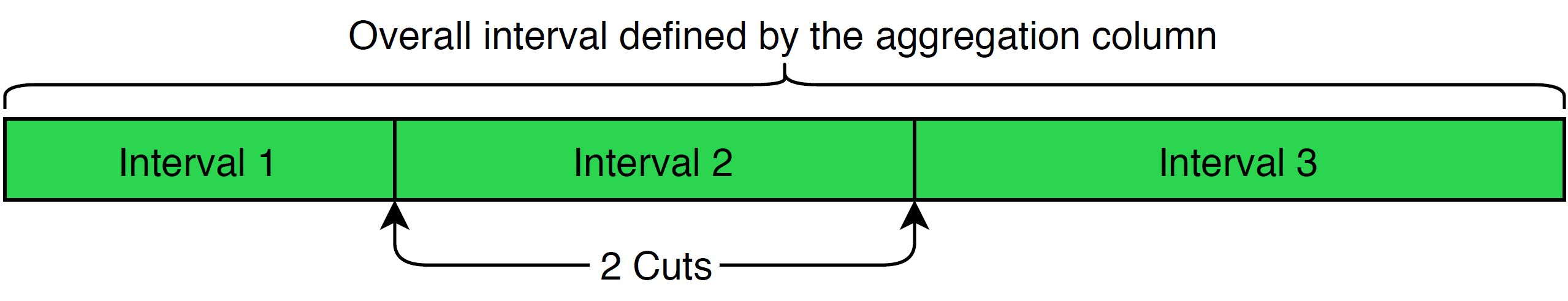## Input

The processor requires a dataset containing a column with timestamps or ratio scaled variables.

## Configuration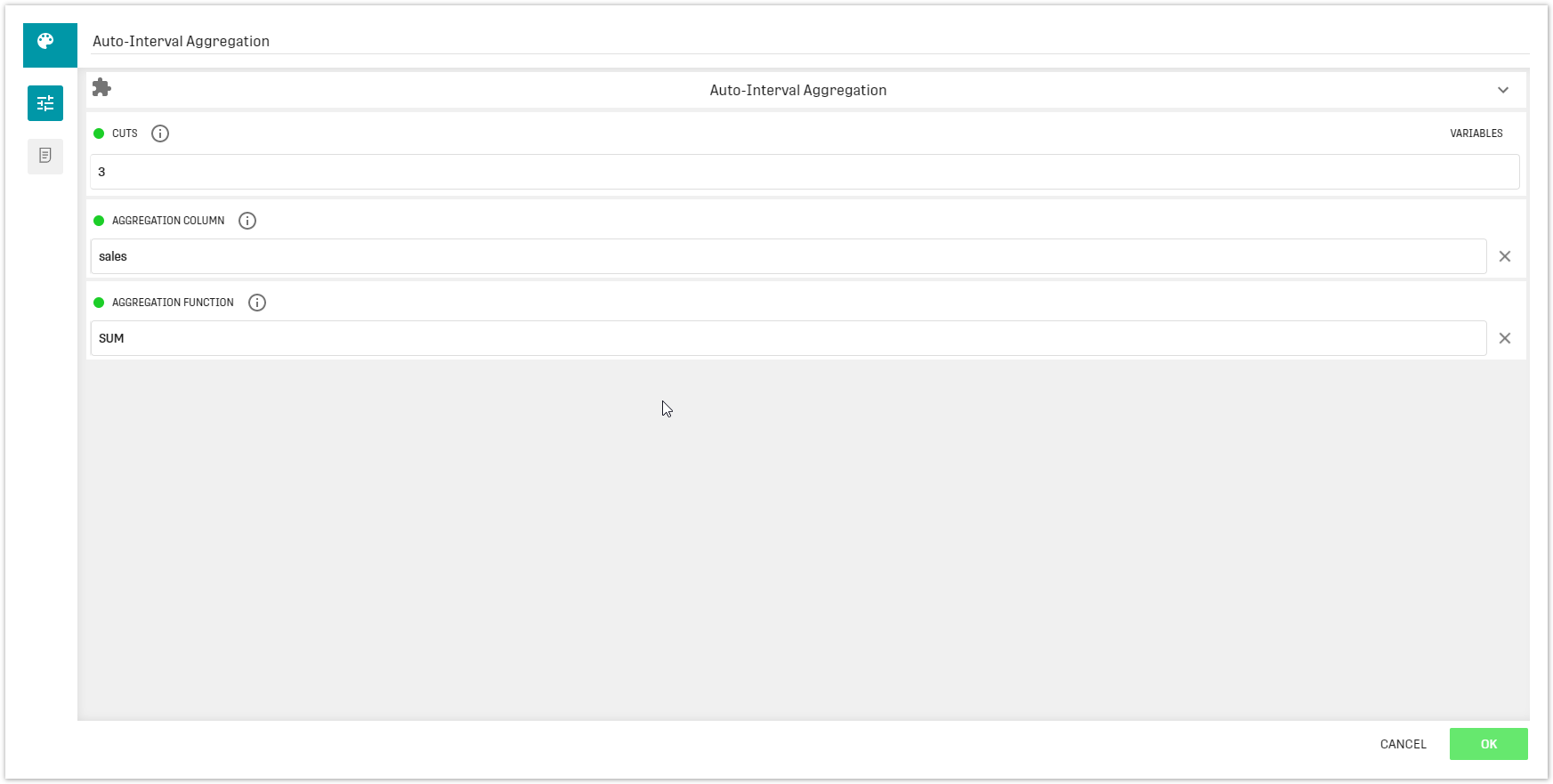Configuration interface

Cuts: Specify the number of cuts and thereby the number of intervals that are produced

Note: There will be one more interval than cuts

Aggregation column: Select the column according to which the intervals are built (e.g. the timestamp column)

Aggregation Function: Select a method (AVG, COUNT, MAX, MIN, SUM) to aggregate the remaining columns in the dataset

Note: Default is set to SUM

## Output

The original dataset cut into several intervals for which the other columns are aggregated.

## Example

In this example only the sales columns from the dataset loaded will be aggregated in the result table.

### Workflow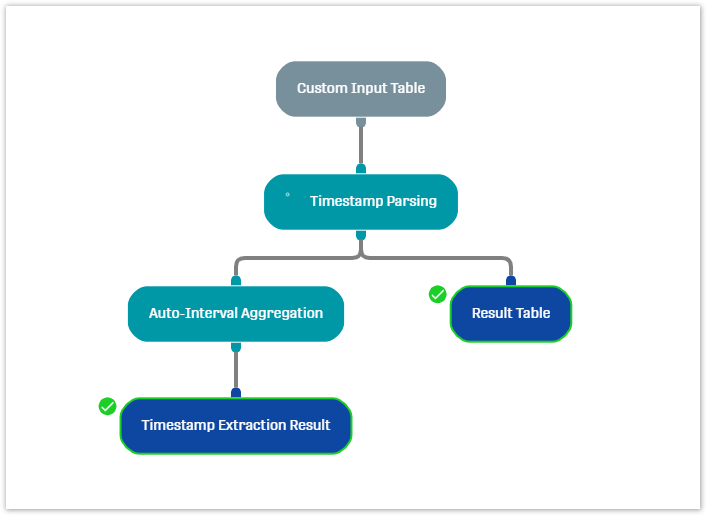### Test data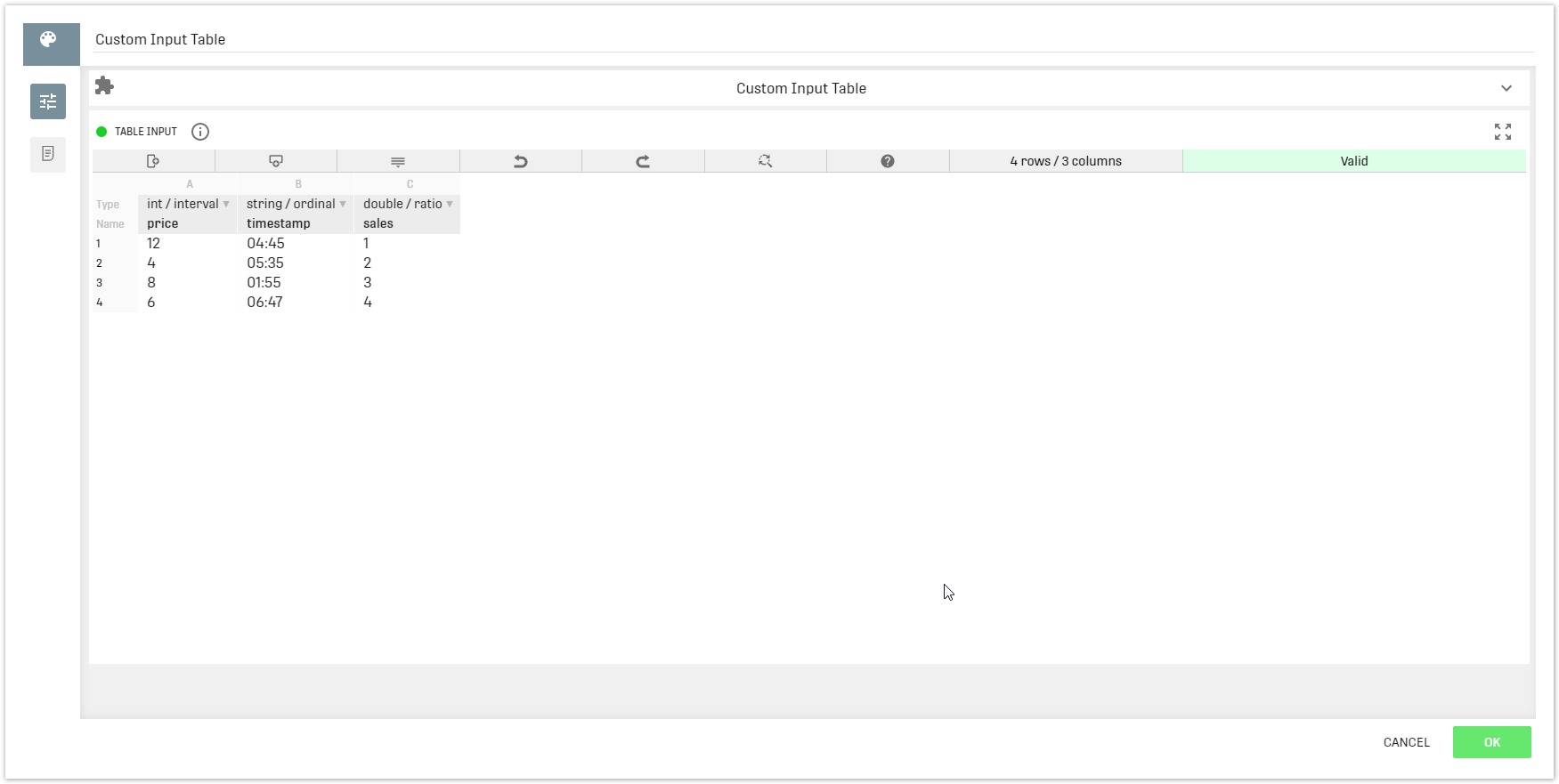Custom Input Table data with timestamp and ratio data

### Result

The table below results from splitting the Custom Input Table data into 2 intervals. The sales column contains the sum of sales for the respective time frames.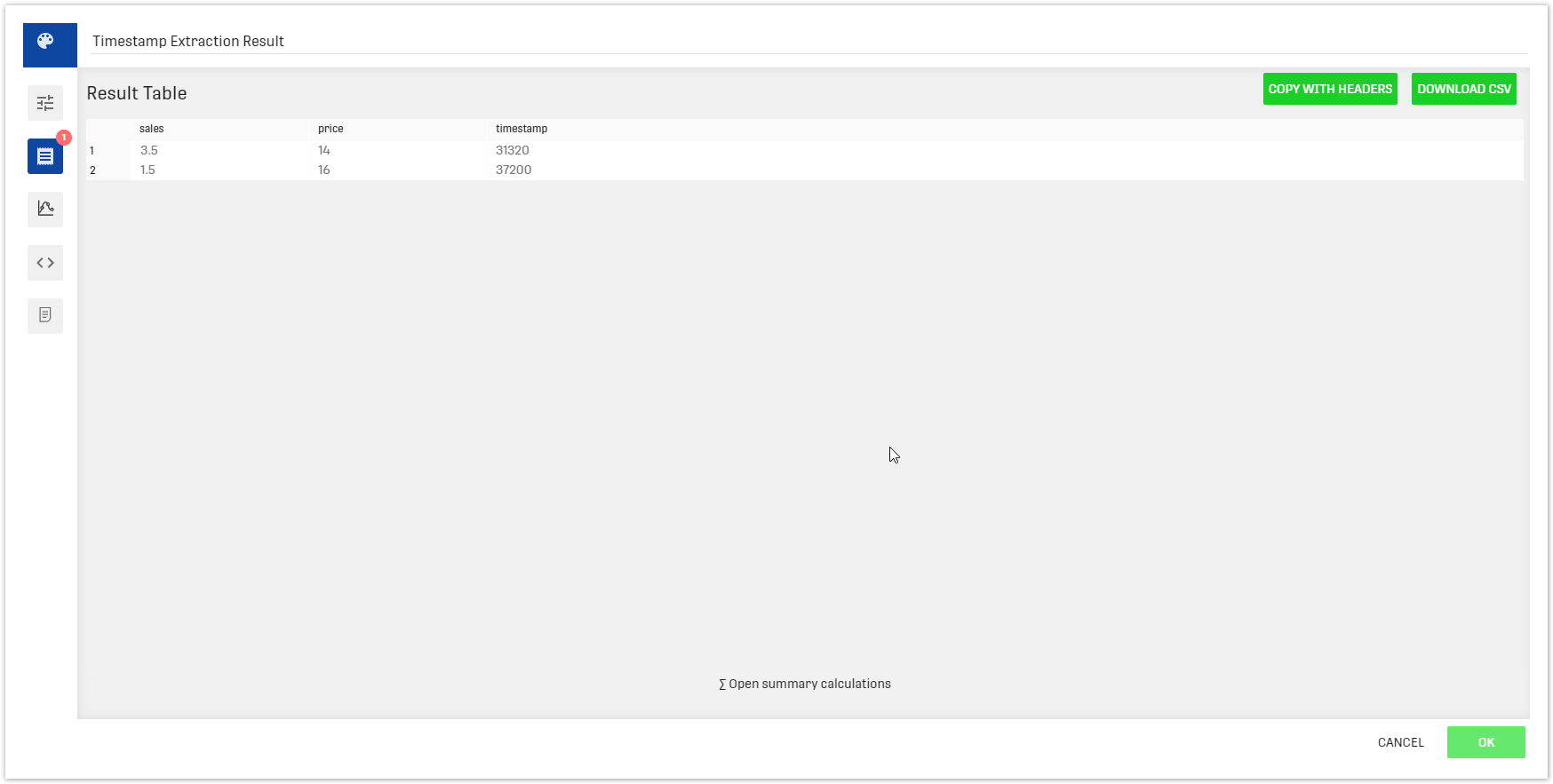Example output